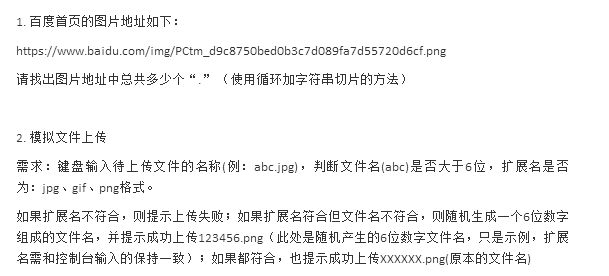2021-09-14 21:15

# 如何使用Python解决如下两道小题（需要详细代码）1.百度首页的图片地址如下：

2.模拟文件上传

• 收藏

#### 1条回答默认 最新

•已采纳

第一个代码

``````url = "https://wwww.baidu.com/xxxxxx.jpg"
s = 0
for i in range(len(url)):
if url[i]==".":
s += 1
print(f"有{s}个'.'")
``````

第二个代码如下：（如有帮助，望采纳！谢谢! 点击我这个回答右上方的【采纳】按钮）

``````import re
import random
fn = input("请输入待上传文件的名称：").strip()
fs,ps = re.findall(r"^(.+)\.(.+)\$",fn)
if ps.lower() in ["jpg","gif","png"]:
if len(fs)>=6:
print("成功上传{}".format(fn))
else:
ds="".join([str(random.randint(0,9)) for i in range(6)])
print("成功上传{}.{}".format(ds,ps))
else:
print("上传失败")
``````
1 打赏 评论